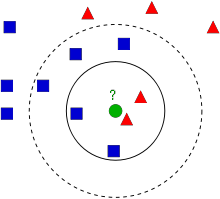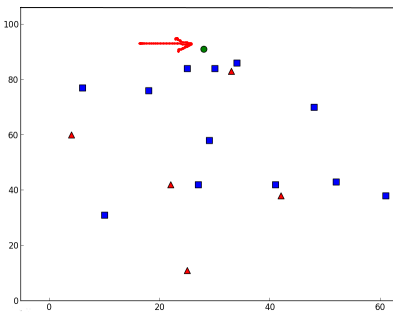# 理解K近邻¶

### 理论¶

kNN是可用于监督学习的最简单的分类算法之一。这个想法是在特征空间中搜索测试数据的最近邻。我们将用下面的图片来研究它。### OpenCV中的kNN¶

import cv2 as cv
import numpy as np
import matplotlib.pyplot as plt
# 包含(x,y)值的25个已知/训练数据的特征集
trainData = np.random.randint(0,100,(25,2)).astype(np.float32)
# 用数字0和1分别标记红色或蓝色
responses = np.random.randint(0,2,(25,1)).astype(np.float32)
# 取红色族并绘图
red = trainData[responses.ravel()==0]
plt.scatter(red[:,0],red[:,1],80,'r','^')
# 取蓝色族并绘图
blue = trainData[responses.ravel()==1]
plt.scatter(blue[:,0],blue[:,1],80,'b','s')
plt.show()


1. 给新样本的标签取决于我们之前看到的kNN理论。如果要使用“最近邻居”算法，只需指定k=1即可，其中k是邻居数。
2. k最近邻的标签。
3. 衡量新加入到每个最近邻的相应距离。

newcomer = np.random.randint(0,100,(1,2)).astype(np.float32)
plt.scatter(newcomer[:,0],newcomer[:,1],80,'g','o')
knn = cv.ml.KNearest_create()
knn.train(trainData, cv.ml.ROW_SAMPLE, responses)
ret, results, neighbours ,dist = knn.findNearest(newcomer, 3)
print( "result:  {}\n".format(results) )
print( "neighbours:  {}\n".format(neighbours) )
print( "distance:  {}\n".format(dist) )
plt.show()


result:  [[ 1.]]
neighbours:  [[ 1.  1.  1.]]
distance:  [[ 53.  58.  61.]]# 10个新加入样本
newcomers = np.random.randint(0,100,(10,2)).astype(np.float32)
ret, results,neighbours,dist = knn.findNearest(newcomer, 3)
# 结果包含10个标签


### 附加资源¶

1. NPTEL关于模式识别的注释，第11章